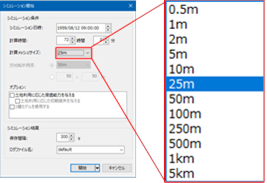# FAQ/ Computational mesh size in latitude-longitude coordinate system¶

DioVISTA uses metric notation for the calculated mesh size, what is [Latitude-longitude coordinate system] the exact length when I select as the coordinate system?

## response¶

DioVISTA uses metric notation for mesh sizes.Figure: [Start Simulation] Dialog

If you select as the coordinate system[Latitude-longitude coordinate system], the mesh used for the calculation is the mesh that is split from the cubic mesh.

The table shows the correspondence between DioVISTA mesh size notation and meshes used for calculation.

Table: Mesh size and number of third-order mesh divisions when latitude-longitude coordinates are selected

Mesh Size (m) Number of third-order mesh divisions
0.5 2000
1 1000
2 500
5 200
10 100
25 40
50 20
100 10
250 4
500 2
1000 1
5000 0.2

For example, a mesh size of 25 m in latitude-longitude coordinates means a mesh that divides one side of a third-order mesh into 40 parts. This mesh size is about 25 m, since the side of the third mesh is about 1 km.

For the exact length of one side of the cubic mesh, see FAQ/What is a standard region mesh? See .

Last update: 2023-03-20